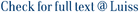Given a set of data graphs and a query graph, graph isomorphism query processing is the problem of finding all the data graphs that are isomorphic to the query graph. Graph isomorphism query processing is a core problem in graph analysis of various application domains. In existing approaches, index construction or query processing takes much time as the graph sizes increase. In this paper, we propose an efficient algorithm for graph isomorphism query processing. We introduce the color-label distribution which represents the canonical coloring of a vertex-labeled graph. Based on degree sequences and color-label distributions, we introduce a two-level index, which helps us efficiently solve graph isomorphism query processing. Experimental results on real datasets show that the proposed algorithm is orders of magnitude faster than the state-of-the-art algorithms in terms of index construction time, and it runs faster than existing algorithms in terms of query processing time as the graph sizes increase.

Efficient Graph Isomorphism Query Processing using Degree Sequences and Color-Label Distributions / Gu, G; Nam, Y; Park, K; Galil, Z; Italiano, Giuseppe Francesco; Han, Ws. - Proceedings - 38th IEEE International Conference on Data Engineering, (2022), pp. 872-884. (IEEE 38th International Conference on Data Engineering (ICDE), Kuala Lumpur, Malaysia, 9-12 May 2022). [10.1109/ICDE53745.2022.00070].

### Efficient Graph Isomorphism Query Processing using Degree Sequences and Color-Label Distributions

#### Abstract

Given a set of data graphs and a query graph, graph isomorphism query processing is the problem of finding all the data graphs that are isomorphic to the query graph. Graph isomorphism query processing is a core problem in graph analysis of various application domains. In existing approaches, index construction or query processing takes much time as the graph sizes increase. In this paper, we propose an efficient algorithm for graph isomorphism query processing. We introduce the color-label distribution which represents the canonical coloring of a vertex-labeled graph. Based on degree sequences and color-label distributions, we introduce a two-level index, which helps us efficiently solve graph isomorphism query processing. Experimental results on real datasets show that the proposed algorithm is orders of magnitude faster than the state-of-the-art algorithms in terms of index construction time, and it runs faster than existing algorithms in terms of query processing time as the graph sizes increase.
##### Scheda breve Scheda completa Scheda completa (DC)2022
978-1-6654-0883-7
graph canonization, canonical coloring, color-label distribution, degree sequence, graph isomorphism query processing
File in questo prodotto:
File
GIQP__ICDE_2022.pdf

Solo gestori archivio

Tipologia: Documento in Pre-print
Licenza: DRM (Digital rights management) non definiti
Dimensione 3.9 MB
Utilizza questo identificativo per citare o creare un link a questo documento: `https://hdl.handle.net/11385/224292`
•0
•0